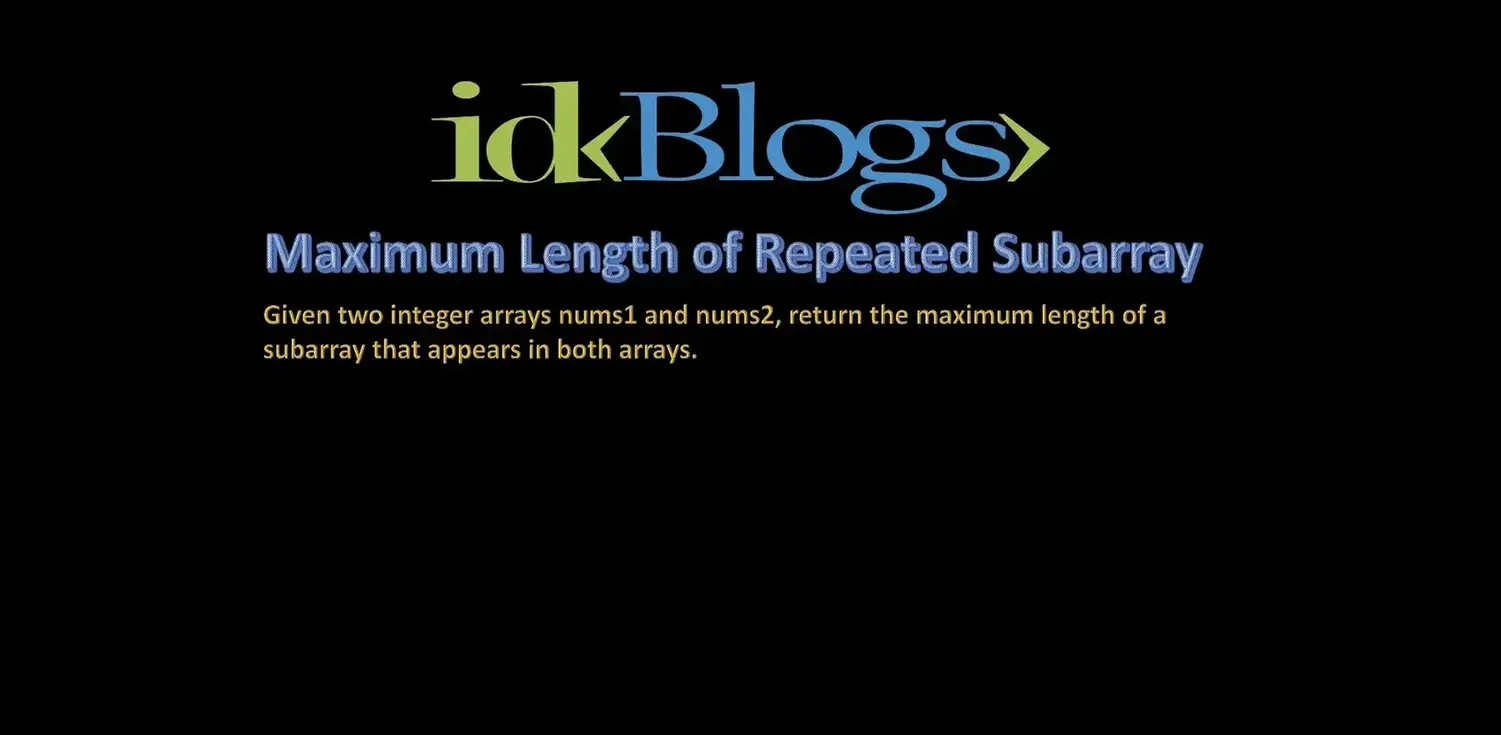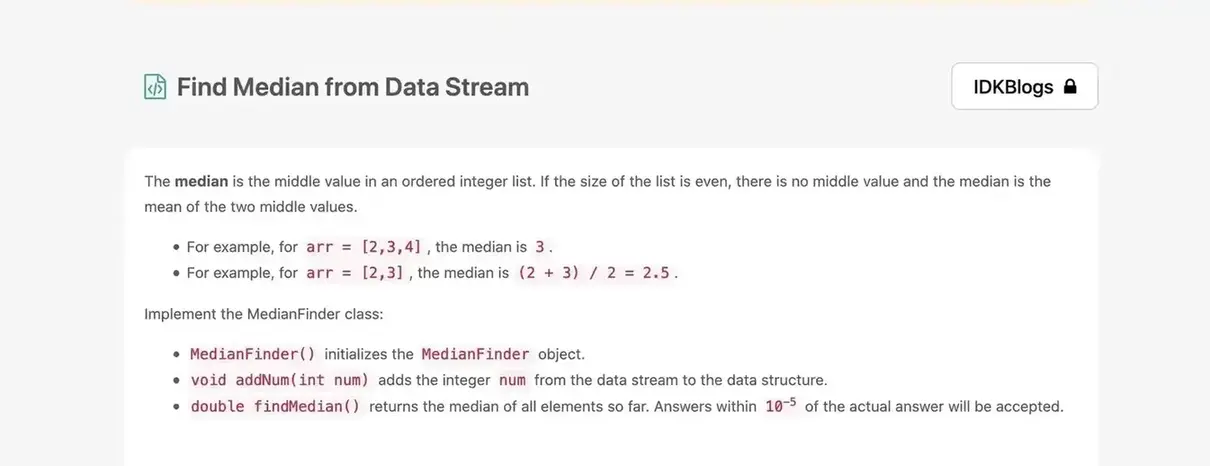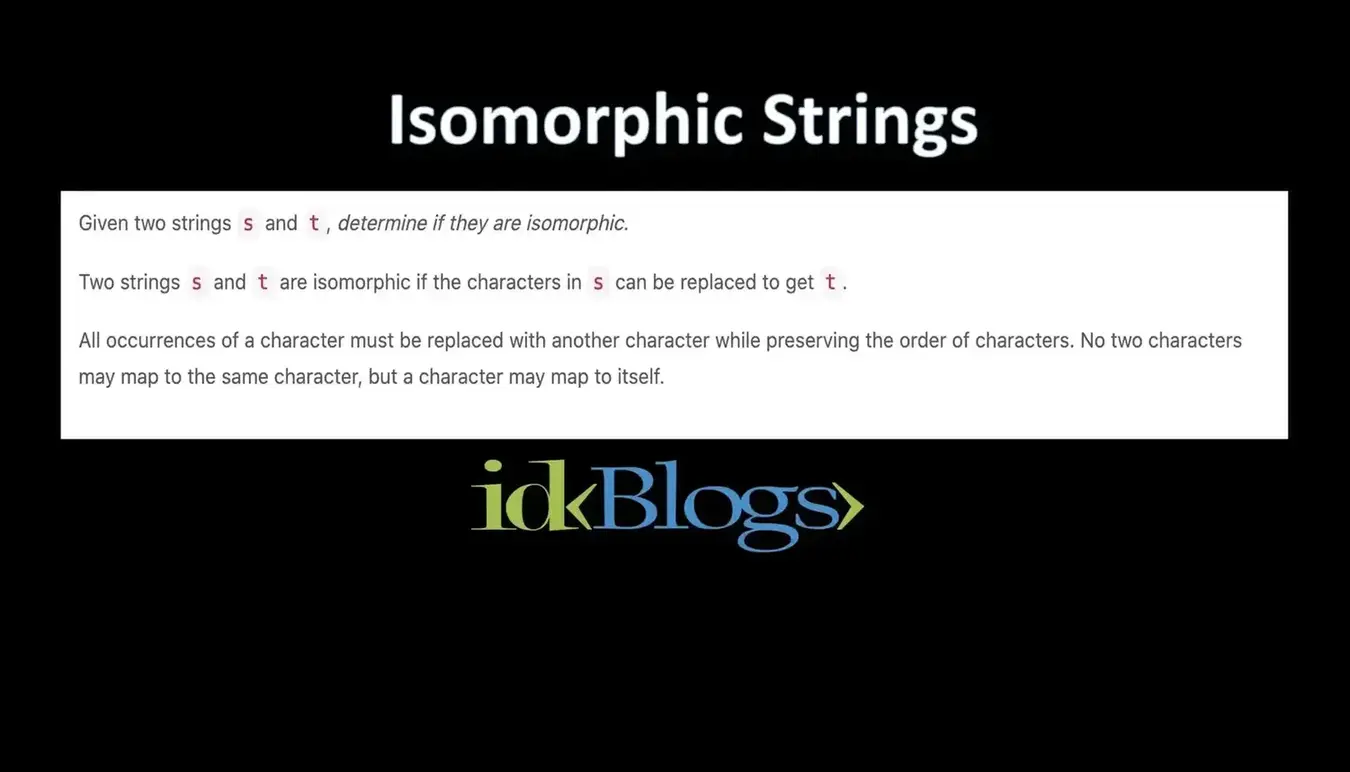# Valid Triangle Number

### Given an integer array nums, return the number of triplets chosen from the array that can make triangles if we take them as side lengths of a triangle.

idkblogs.com      July 27, 2021Shubham Verma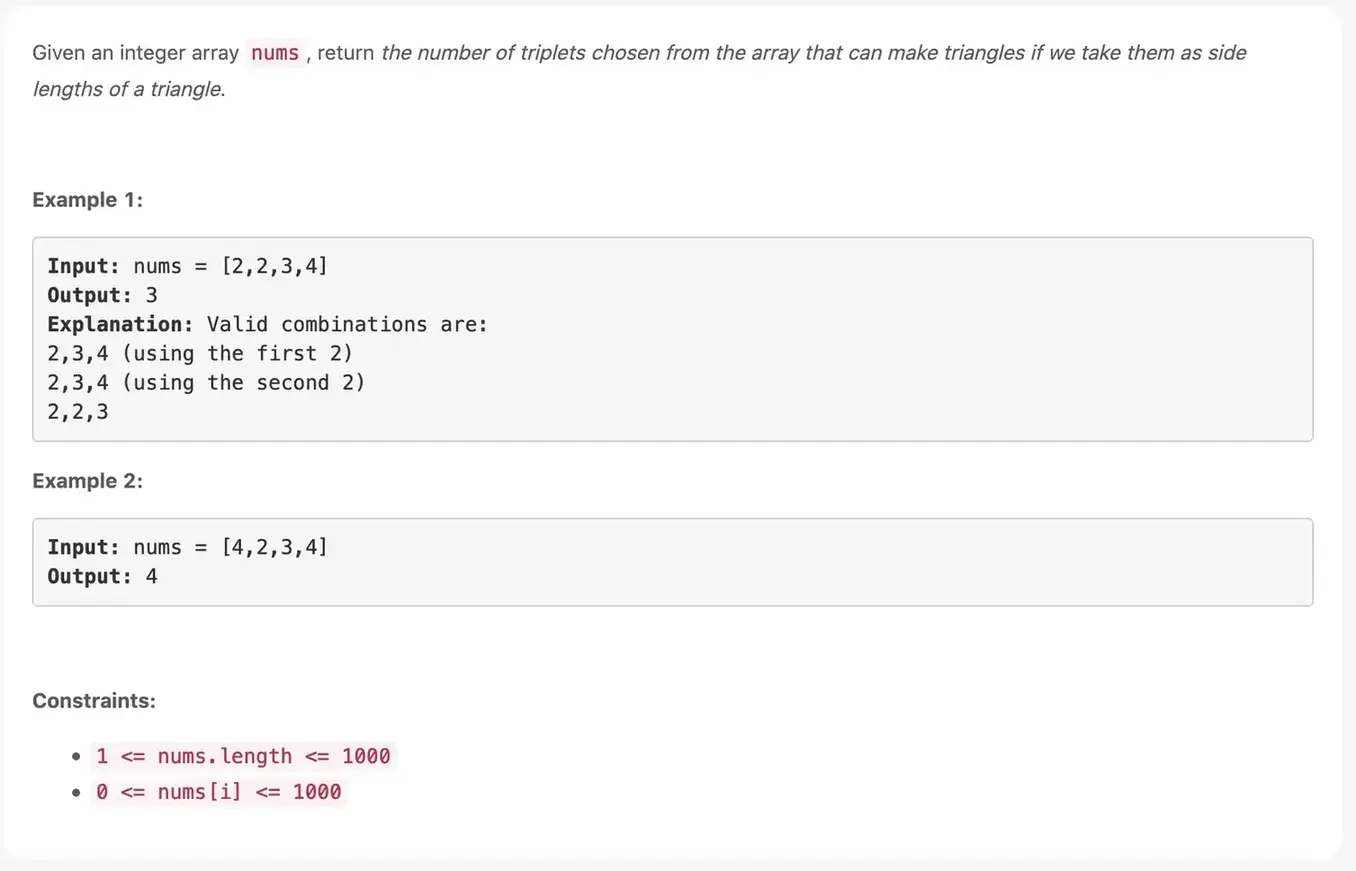# Valid Triangle Number:

Given an integer array nums, return the number of triplets chosen from the array that can make triangles if we take them as side lengths of a triangle.

# Example 1:

Input: nums = [2,2,3,4]
Output: 3

# Explanation: Valid combinations are:

2,3,4 (using the first 2)
2,3,4 (using the second 2)
2,2,3

# Example 2:

Input: nums = [4,2,3,4]
Output: 4

# Constraints:

1 <= nums.length <=1000
0 <= nums[i] <=1000

# Solution:

``````                               /**
* @param {number[]} nums
* @return {number}
*/

var triangleNumber = function(arr) {
// Count of triangles
let count = 0;
let n = arr.length;

// The three loops select three
// different values from array
for (let i = 0; i < n; i++) {
for (let j = i + 1; j < n; j++) { // The innermost loop checks for // the triangle property
for (let k = j + 1; k < n; k++) // Sum of two sides is greater // than the third if ( arr[i] + arr[j]> arr[k]
&& arr[i] + arr[k] > arr[j]
&& arr[k] + arr[j] > arr[i])
count++;
}
}
return count;
};
``````

# Output: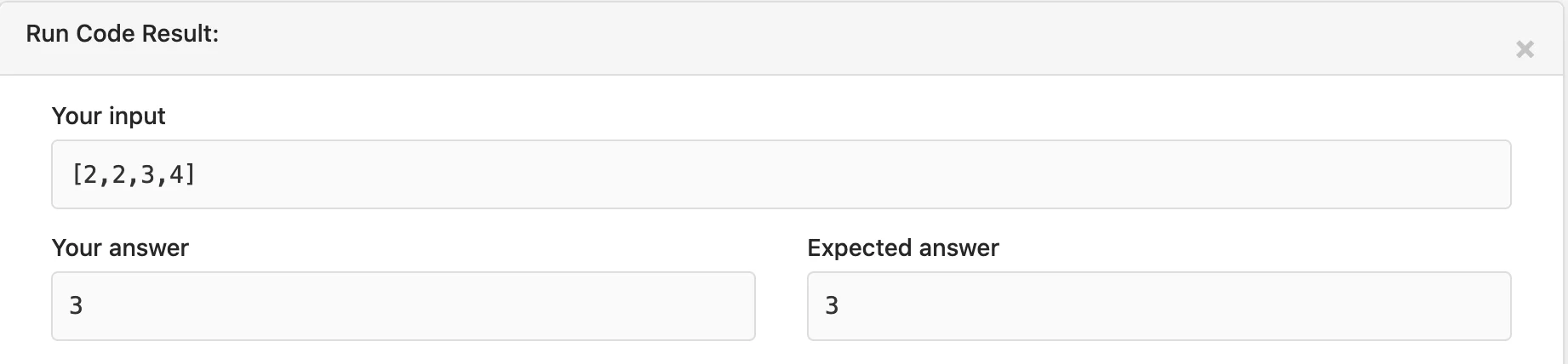Valid Triangle Number

Thank you

To read more about the technologies, Please subscribe us, You'll get the monthly newsletter having all the published article of the last month.

### OTHER POSTS